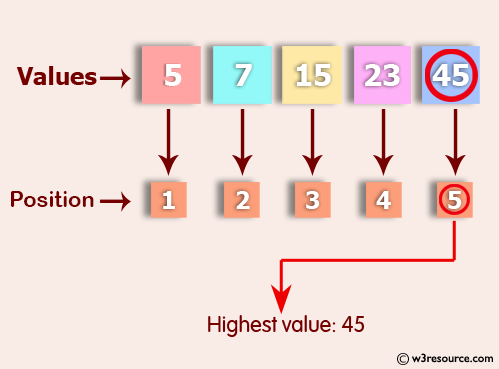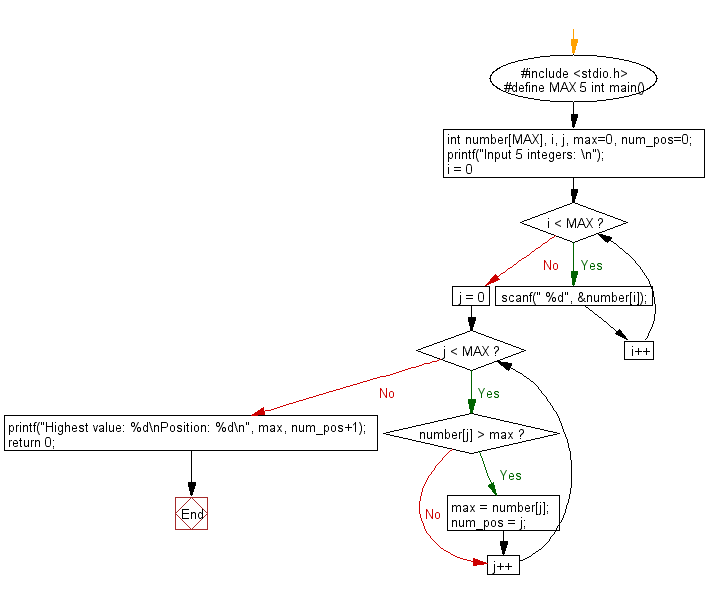﻿ C : The highest integer and its position from some integers

# C Exercises: Accepts some integers from the user and find the highest value and the input position

## C Basic Declarations and Expressions: Exercise-33 with Solution

Write a C program that accepts some integers from the user and finds the highest value and the input position.

Pictorial Presentation:C Code:

``````#include <stdio.h>
#define MAX 5
int main()
{
int number[MAX], i, j, max=0, num_pos=0; // Declare an array to store 5 numbers, and variables for loop counters and maximum value
printf("Input 5 integers: \n");

for(i = 0; i < MAX; i++) {
scanf(" %d", &number[i]); // Read 5 integers from the user
}

for(j = 0; j < MAX; j++)
{
if(number[j] > max) { // Check if current number is greater than current maximum
max = number[j]; // Update maximum if needed
num_pos = j; // Record the position of maximum
}
}

printf("Highest value: %d\nPosition: %d\n", max, num_pos+1); // Print the maximum value and its position
return 0;
}
``````

Sample Output:

```Input 5 integers:
5
7
15
23
45
Highest value: 45
Position: 5
```

Flowchart:C Programming Code Editor:

What is the difficulty level of this exercise?

Test your Programming skills with w3resource's quiz.

﻿